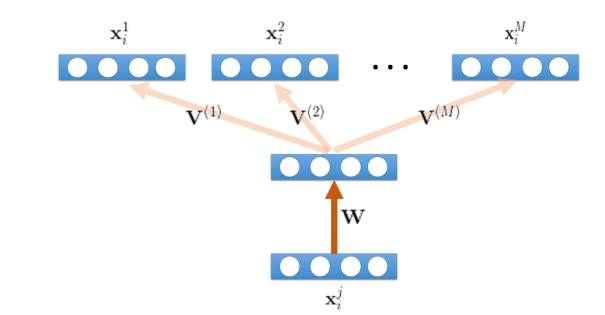# Autoencoder with multiple decoders

Dear Team, I am trying to create an auto-encoder model which has one encoder and multiple decoders(depending on the number of classes). I am stuck while optimizing the loss. The loss is calculated by adding all the losses of the decoder output and the original data matrix for each class. I am not getting how to get it combinedly for all the classes together. Some insights will be helpful. Thanks

``````import torch
import torch.nn as nn
from dataset import Dataset
from autoencoder import Autoencoder
import numpy as np
``````

device = torch.device(“cuda:0” if torch.cuda.is_available() else “cpu”)

num_epochs = 500
batch_size = 50
learning_rate = 0.01

model = Autoencoder().to(device)
criterion = nn.MSELoss()
def init(self):

self.ec = nn.Linear(29,50)
self.dc1= nn.Linear(50,29)
self.dc2= nn.Linear(50,29)
self.dc3 = nn.Linear(50,29)
self.dc4= nn.Linear(50,29)
self.dc5= nn.Linear(50,29)``````def forward(self, x, dom=0):
x = self.ec(x)
out1 = self.dc1(x)
# print ("out1:", out1.shape)

out2 = self.dc2(x)
#print ("out2:", out2.shape)

out3 =self.dc3(x)
#print ("out3:", out3.shape)
#out4 = self.dc4(x)
# out5 = self.dc5(x)
final_out = torch.vstack((out1, out2, out3))

return final_out
``````

# print(out.shape)

def train_AE():
dataset = Dataset()

``````for epoch in range(100):
train_loss = 0.0

input = input.float().to(device)

#print('input.shape = ', input.shape)  # 128 * 256
dom_out = out.float().to(device) ## original data # 128 * 256
#print('dom_out.shape = ', dom_out.shape) # 128 * 256

#print('predicted output.shape = ', output.shape) # 128 * 256 -- reconstructed values

out1 = output[0:50,:]
out2 = output[50:100,:]
out3 = output[100:150,:]

loss1 = criterion(out1, dom_out)
loss2 = criterion(out2,dom_out) # wrong
loss3 = criterion(out3,dom_out)

final_loss = loss1 + loss2 + loss3

final_loss.backward()